Courses

# Solved Examples - Exponents & Roots GMAT Notes | EduRev

## Quantitative Aptitude for SSC CGL

Created by: Ria Khurana

## GMAT : Solved Examples - Exponents & Roots GMAT Notes | EduRev

The document Solved Examples - Exponents & Roots GMAT Notes | EduRev is a part of the GMAT Course Quantitative Aptitude for SSC CGL.
All you need of GMAT at this link: GMAT

Section - 1
Simplify the following expressions by combining like terms. If the base is a number, leave the answer in exponential form (i.e. 23, not 8).

Ques 1. x5 x x3
Ans:
x5 x x3 = x(5+3= x8

Ques 2: 76 x 7
Ans:
76 x 79 = 7(6+9) = 715

Ques 3: 55/55
Ans: 5(5-3) = 52

Ques 4: (a3)2
Ans:
(a2)3 = a(3x2) = a6

Ques 5: 4-2 x 4
Ans:
4-2 x 45 = 4(-2+5) = 43

Ques 6: (-3)a/(-3)2
Ans:  (-3)(a-2)

Ques 7: (3)2-3
Ans:
(32)-3 = 3(2 x -3) = 3-6

Ques 8: 114/11x
Ans: 11(4-x)

Ques 9:  x2 x x3 x x5
Ans:
x2 x x3 x x= X (2 + 3 + 5) = x10

Ques 10: (52)x
Ans: (52)x=5(2 * x)= 52x

Section - 2
Ques 11: 34 x 3x 3

Ans: 34 x 32 x 3
= 3(4 + 2 + 1) = 37

Ques 12: xx x6 /x2
Ans: x(5+6- 2) = x9

Ques 13:  56 x 54x / 54
Ans:
5(6+ 4x—4) = 54x+2

Ques 14: y7 x y8 x y-6
Ans: yx y8 x y-6
= y(7 + 8 + (-6))
= y9

Ques 15: x4/x-3
Ans: x(4-(-3)) = x7

Ques 16: z5 x z-3/z-8
Ans: z(5+(-3)-(-8))= z10

Ques 17: 32x x 36x/3-3y
Ans: 3(2x+6x-(-3y)) = 38x+3y

Ques 18: (x2)6 x x3
Ans:
x(2 x 6 + 3) = x(12+3) = x15

Ques 19: (z6)x x z3x
Ans:
z(6 * x+3x) = z(6x+3x) = z9x

Ques 20: 53 x (54)y/(5y)3
Ans:
5(3+(4xy)-(y x 3)) = 5(3+4y-3y)=  5y+3

Section - 3
Ques 21: Compute the sum.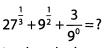Ans: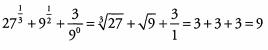Ques 22: Which of the following has the lowest value?
(A) (-3)4

(B) -33
(C) (-3)-3
(D) (-2)3
(E) 2-6
Ans: We are looking for the answer with the lowest value, so we can focus only on answers that are negative as these answers have lower values than any positive answers.
(A) (-3)4 will result in a positive number because 4 is an even power.
(B) -33 = -(3)3 = -27
The exponent is done before multiplication (by -1) because of the order of operations.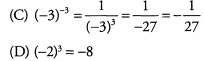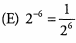The value of this expression is positive.
-2 7 has the lowest value of the three answer choices that result in negative numbers. The correct answer is (B).

Ques 23: Compute the sum.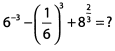Ans: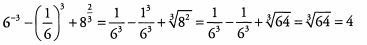The first two terms in the expression are in fact the same. Because these terms are equal, when the second is subtracted from the first they cancel out leaving only the third term.

Ques 24: Which of the following is equal to (2/5)-3?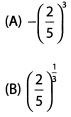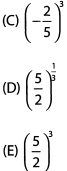Ans: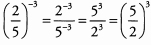Note: when a problem asks you to find a different or more simplified version of the same thing, check your work against the answer choices frequently to ensure that you don’t simplify or manipulate too far.

Ques 25: Which of the following has a value less than 1 ? (Select all that apply)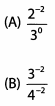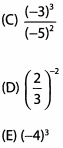Ans:
We are looking for values less than 1 so any expressions with negative values, zero itself, or values between 0 and 1 will work.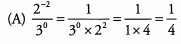Dividing a smaller positive number by a larger positive number will result in a number less than 1.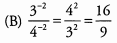Dividing a larger positive number by a smaller positive number will result in a number greater than 1.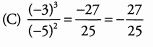This answer is negative; therefore, it is less than 1.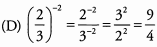Dividing a larger positive number by a smaller positive number will result in a number greater than 1.
(E) (-4)3 = -64
This answer is negative; therefore, it is less than 1.

Section - 4
Simplify the following expressions by finding common bases.

Ques 26: 83 x 26
Ans: 83 x 26 = (23)3 x 26 = 29 x 26 = 215

Ques 27: 492 x 77
Ans: (72)2 x 77 = 74 x 77 = 711

Ques 28: 254 x 1253
Ans:
(52)4 x (53)3 = 58 x 59 = 517

Ques 29: 9-2 x 272
Ans:
(32)-2 x (33)2 = 3-4 x 36 = 32

Section - 5
Simplify the following expressions by pulling out as many common factors as possible.

Ques 31: 63 + 33 = (A) 35 (B) 39 (C) 2(33)
Ans:
Begin by breaking 6 down into its prime factors.
63 + 33 =
(2 x 3)3 + 33 =
(23) (33) + 33
Now each term contains (33). Factor it out.
(23)(33) + 33 =
33(23 + 1) =
33(9) =
33(32) = 35
We have a match. The answer is A.

Ques 32: 813 + 274 = (A) 37(2) (B) 312(2) (C) 314
Ans:
Both bases are powers of 3. Rewrite the bases and combine.
813 + 274 =
(34)+ (33)4 =
312 + 312 =
312(1 + 1) =
312(2)
We have a match. The answer is B.

Ques 33: 152- 52 = (A) 52(2) (B) 5223 (C) 5232
Ans:
Begin by breaking 15 down into its prime factors.
152 - 52 =
(3 x 5)2 - 52 =
(32)(52) - 52
Now both terms contain 52. Factor it out.
(32)(52) - 52 =
52(32 - 1) =
52(9 - 1) =
52(8)
We still don’t have a match, but we can break 8 down into its prime factors.
52(8) =
52(23)
We have a match. The answer is B.

Section 6
Ques 34: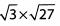Ans: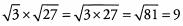Ques 35: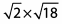Ans: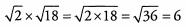Ques 36: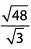Ans: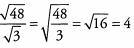Ques 37: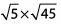Ans: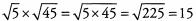Ques 38: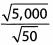Ans: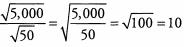Ques 39: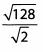Ans: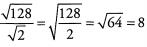Ques 40: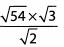Ans: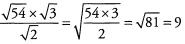Ques 41: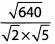Ans: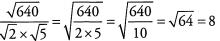Ques 42: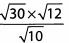Ans: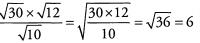Section - 7
Simplify the following roots. Not every answer will be an integer.
Ques 43: √32
Ans: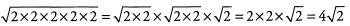Ques 44: √24
Ans: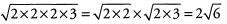Ques 45: √180
Ans: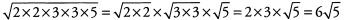Ques 46: √490
Ans: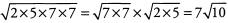Ques 47: √450
Ans: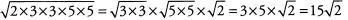Ques 48: √135
Ans: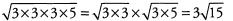Ques 49: √224
Ans: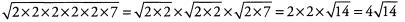Ques 50: √343
Ans: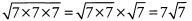Section - 8
Simplify the following roots. You will be able to completely eliminate the root in every question. Ex- press answers as integers.
Ques 51: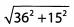Ans: Pull out the greatest common factor of 362 and 152, namely 32, to give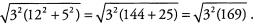Both 32 and 169 are perfect squares (169 = 132), so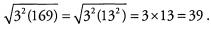Ques 52: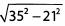Ans: Pull out the greatest common factor of 352 and 212, namely 72, to give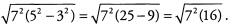Both 72 and 16 are perfect squares (16 = 42), so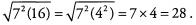Ques 53: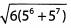Ans: Pull out the greatest common factor of 56 and 57, namely 56, to give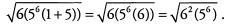Both 62 and 5are perfect squares (56= 5x 53), so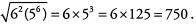Ques 54: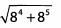Ans: Pull out the greatest common factor of 84 and 85, namely 84, to give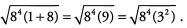Both 84 and 32 are perfect squares (84 = 82 x 82), so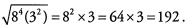Ques 55: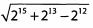Ans: Pull out the greatest common factor of 215, 213, and 212, namely 212, to give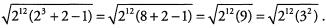Both 212 and 32 are perfect squares

(212 = 26 x 26),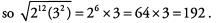Ques 56: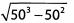Ans: Pull out the greatest common factor of 503 and 502, namely 502, to give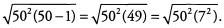Both 502 and 72 are perfect squares, so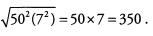Offer running on EduRev: Apply code STAYHOME200 to get INR 200 off on our premium plan EduRev Infinity!

,

,

,

,

,

,

,

,

,

,

,

,

,

,

,

,

,

,

,

,

,

;